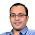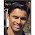### Math Olympiad Problem : Overlapping Coins

Source: On Quora

Problem: A rectangular table has 100 coins placed on it (centers must be on the table) such that none of the coins overlap, and it is impossible to place any more coins on the table without causing an overlap.
Prove that using 400 coins and allowing overlaps, we can cover the entire table.

Update (2/2/2013):
Assumptions:
1) Coins are identical and round.
2) You are given a configuration of 100 coins satisfying the first condition. You are to prove that there exists a fresh configuration of 400 coins satisfying the second condition.
3) Covering the table means for all points on the table, there is at least one coin above that point
4) Coins placed on the table means that the center of the coin is on the table

Solution posted by Stephen Rong in comments!

1.Are all coins same size?

Can coins be layered like fish scales (such that coin edges matter)?

Can first 100 coins be assumed as placed on their edges?

Is this a mathematical problem (assume perfect circles) or a practical problem (assume imperfect coins)?

Does friction matter (can we provide an unstable solution that might solve for a very short time)?

Can I use non-circular coins?

Should the solution require at least 400 coins (i.e. answer must set up initial state such that 400 is minimum for solution?)

Etc.Etc.

1.@Mo Jons..
Are you dumb? Do you even want to solve the problem? Why don't you melt the coins first? Why didn't you ask if we were on earth or on a different planet? Are we talking in english because coins might mean something else in a different language? Define imperfect coins! Seriously, do people in your world specify friction in all the math problems? Get out of here!

2.Ambiguity in problem: Even assuming that coins are round and identical, do u mean any one particular arangement or any arrangement that fits the bill? Also does the original arrangement of 100 coins need to be preserved in the final array?

Considering the worst case scenario:
If the 10 coins are arrange in a hexagonal array (not a close-packed one) such that in each equilateral triangle formed by the three nearest coins, the distance from the centroid to the edge of the coin is just smaller than the coin radius, then such an arrangement meets the requirement of the problem. As it turns out, overlapping coin will be just greater than the incircle.

Changing the original array in the final arrangement:
The area of the table is (6*sqrt3/pi)*area of 100 coins or roughly 3.308 times the area of all coins put together. If the area of a coin is taken as 1 unit, the area of the table is 330.8 units
So theoretically, 400 coins should seem more than sufficient. However, there is the issue of the circular geometry.

Using a square packing arrangement (78.54% packing fraction), the base layer uses 260 coins with an additional layer of 260 coins required to cover up the rest. total = 520.

Using hexagonal packing requires 900 coins (3 layers of 300 coins, not giving the calculations here).

Can't see how a regular packing arrangement can occupy 100% space in just 400 coins.

1.This is a standard Maths Olympiad problem. Its obvious to assume that coins are round and identical. If you try hard enough, you will always find a way not to solve a problem. Unfortunately, we are trying to solve the problem.

3.Just a small clarification in the previous post:

When I said worst case scenario, I meant spacing out the coins to the maximum, in an arrangement that minimized table area as larger table area will obviously need more coins to cover.

4.Very very nice problem!

Solution: I'll talk about circles instead of coins. Let the circles have radius 1. 100 of these circles are distributed on a rectangle, no two coins overlap, and no more coins can be placed on the table.

Consider just one of these circles, with center P. It follows that the center Q of any other circle cannot lie within the circle of radius 2 with center P because it must be at least 2 units away. Thus, we construct all of these circles of radius 2, concurrent with each of the circles of radius 1. If the set of circles of radius 2 did not cover the rectangle entirely, then we could place a circle of radius 1 in this region, contradiction. Thus, the set of circles of radius 2 entirely covers the rectangle.

We now have 100 circles of radius 2 that entirely covers the rectangle. Scale this by a factor of 1/2 in both planar dimensions. Now we have 100 circles of radius 1 that entirely covers a rectangle that is a quadrant of the original rectangle. By placing four of these sets together, we get 400 circles of radius 1 that entirely covers the original rectangle. QED

1.Great solution Stephen. Thanks a ton.

2.this is old, but the point is that if there's a spot on the table that isn't covered by the circles of radius 2, then you could put a circle of radius 1 centered at that spot on the original table, and it wouldn't overlap with any of the existing circles. This is because the circles of radius 2 cover every point that's within a distance 1 of any original circle.